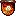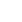<% FormBG = "bgcolor = " & chr(34) & "#000000" & chr(34) FormScope = "/" shmackver = "3.5" SiteLocale = "EN-US" %> >
<% ' Set Initial Conditions NewQuery = TRUE UseSavedQuery = FALSE SearchString = "" QueryForm = Request.ServerVariables("PATH_INFO") ' Did the user press a SUBMIT button to execute the form? If so get the form variables. if Request.ServerVariables("REQUEST_METHOD") = "POST" then SearchString = Request.Form("SearchString") PageSize = Request.Form("PageSize") SortBy = Request.Form("SortBy") Order = Request.Form("Order") ' NOTE: this will be true only if the button is actually pushed. if Request.Form("Action") = "Search" then NewQuery = TRUE RankBase=1000 end if end if if Request.ServerVariables("REQUEST_METHOD") = "GET" then SearchString = Request.QueryString("qu") PageSize = Request.Form("PageSize") SortBy = Request.Form("SortBy") Order = Request.Form("Order") FormScope = Request.QueryString("sc") RankBase = Request.QueryString("RankBase") if Request.QueryString("pg") <> "" then NextPageNumber = Request.QueryString("pg") NewQuery = FALSE UseSavedQuery = TRUE else NewQuery = SearchString <> "" end if end if %>

Enter ARTIST or SONG NAME below. For a full list enter MP3.

 5 10 15 25 50 75 100 250 Date Name Size Descending Ascending
<% if NewQuery then set Session("Query") = nothing set Session("Recordset") = nothing set Session("PageSize") = nothing set Session("SortBy") = nothing 'set Session("Order") = nothing NextRecordNumber = 1 ' Remove any leading and ending quotes from SearchString SrchStrLen = len(SearchString) if left(SearchString, 1) = chr(34) then SrchStrLen = SrchStrLen-1 SearchString = right(SearchString, SrchStrLen) end if if right(SearchString, 1) = chr(34) then SrchStrLen = SrchStrLen-1 SearchString = left(SearchString, SrchStrLen) end if if FreeText = "on" then CompSearch = "\$contents " & chr(34) & SearchString & chr(34) else CompSearch = "@filename " & SearchString & chr(42) end if set Q = Server.CreateObject("ixsso.Query") set util = Server.CreateObject("ixsso.Util") Q.Query = CompSearch Q.SortBy = SortBy & Order Q.Columns = "DocTitle, vpath, filename, size, write, rank" Q.MaxRecords = 100000 if FormScope <> "/" then util.AddScopeToQuery Q, FormScope, "deep" end if if SiteLocale<>"" then Q.LocaleID = util.ISOToLocaleID(SiteLocale) end if set RS = Q.CreateRecordSet("nonsequential") RS.PageSize = PageSize ActiveQuery = TRUE elseif UseSavedQuery then if IsObject( Session("Query") ) And IsObject( Session("RecordSet") ) And IsObject( Session("PageSize") ) And IsObject( Session("SortBy") ) then set Q = Session("Query") set RS = Session("RecordSet") set PS = Session("PageSize") set SB = Session("SortBy") if RS.RecordCount <> -1 and NextPageNumber <> -1 then RS.AbsolutePage = NextPageNumber NextRecordNumber = RS.AbsolutePosition end if ActiveQuery = TRUE else Response.Write "ERROR - No saved query" end if end if if ActiveQuery then if not RS.EOF then %>

<% LastRecordOnPage = NextRecordNumber + RS.PageSize - 1 CurrentPage = RS.AbsolutePage if RS.RecordCount <> -1 AND RS.RecordCount < LastRecordOnPage then LastRecordOnPage = RS.RecordCount end if Total = SortBy & Order Response.Write "Songs " & NextRecordNumber & " to " & LastRecordOnPage if RS.RecordCount <> -1 then Response.Write " of " & RS.RecordCount end if Response.Write " matching the query " & chr(34) & "" Response.Write SearchString & "" & chr(34) & ". Search results are listed by " & Total & "" %>

<% if Not RS.EOF and NextRecordNumber <= LastRecordOnPage then%> <% end if %> <% Do While Not RS.EOF and NextRecordNumber <= LastRecordOnPage ' This is the detail portion for Title, Abstract, URL, Size, and ' Modification Date. ' If there is a title, display it, otherwise display the filename. %> <% RS.MoveNext NextRecordNumber = NextRecordNumber+1 Loop %>
 <% ' Graphically indicate rank of document with list of stars (*'s). if NextRecordNumber = 1 then RankBase=RS("rank") end if if RankBase>1000 then RankBase=1000 elseif RankBase<1 then RankBase=1 end if NormRank = RS("rank")/RankBase if NormRank > 0.90 then stars = "rankbtn5.gif" elseif NormRank > 0.75 then stars = "rankbtn4.gif" elseif NormRank > 0.60 then stars = "rankbtn3.gif" elseif NormRank >.50 then stars = "rankbtn2.gif" else stars = "rankbtn1.gif" end if %> <%= NextRecordNumber%>. <%if VarType(RS("DocTitle")) = 1 or RS("DocTitle") = "" then%> " class="RecordTitle"><%= Server.HTMLEncode( RS("filename") )%> <%else%> " class="RecordTitle"><%= Server.HTMLEncode(RS("DocTitle"))%> <%end if%>" class="genrow2" style="color:darkblue;"> <%if RS("size") = "" then%>(size and time unknown)<%else%>size <%=RS("size")%> bytes - created <%=RS("write")%> <%end if%>

<% else ' NOT RS.EOF if NextRecordNumber = 1 then Response.Write "No songs matched that query

" else Response.Write "No songs matched that query

" end if end if ' NOT RS.EOF if NOT Q.OutOfDate then ' If the index is current, display the fact %> The index is up to date.
<%end if if Q.QueryIncomplete then ' If the query was not executed because it needed to enumerate to ' resolve the query instead of using the index, but AllowEnumeration ' was FALSE, let the user know %>

The query is too expensive to complete.
<%end if if Q.QueryTimedOut then ' If the query took too long to execute (for example, if too much work ' was required to resolve the query), let the user know %>

The query took too long to complete.
<%end if%> <% ' This is the "previous" button. ' This retrieves the previous page of documents for the query. %> <%SaveQuery = FALSE%> <%if CurrentPage > 1 and RS.RecordCount <> -1 then %> <%SaveQuery = TRUE%> <%end if%> <% ' This is the "next" button for unsorted queries. ' This retrieves the next page of documents for the query. if Not RS.EOF then%> <%SaveQuery = TRUE%> <%end if%>
 <% NextString = "Next " if RS.RecordCount <> -1 then NextSet = (RS.RecordCount - NextRecordNumber) + 1 if NextSet > RS.PageSize then NextSet = RS.PageSize end if NextString = NextString & NextSet & " songs" else NextString = NextString & " page of songs" end if %>
Displaying Results Page <% ' Display the page number %> <%=CurrentPage%> <%if RS.PageCount <> -1 then Response.Write "of " & RS.PageCount & "." end if %> <% ' If either of the previous or back buttons were displayed, save the query ' and the recordset in session variables. if SaveQuery then set Session("Query") = Q set Session("RecordSet") = RS 'set Session("PageSize") = PS 'set Session("SortBy") = SB else RS.close Set RS = Nothing Set Q = Nothing set Session("Query") = Nothing set Session("RecordSet") = Nothing 'set Session("PageSize") = Nothing 'set Session("SortBy") = Nothing end if %> <% end if %>Shmack! MP3 search version <%=shmackver%>MP3 searches since 6/23/2000